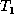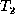Next: Outline of Algorithm Up: Change Detection in Previous: A Cost Model

# Generating the Edit Script

In this section we consider the Minimum Conforming Edit Script problem, motivated in the previous section. The problem is stated as follows. Given a tree(the old tree), a tree(the new tree), and a (partial) matching M between their nodes, generate a minimum cost edit script that conforms to M and transformsto. Our algorithm starts with an empty edit script E and appends edit operations to E as it proceeds. To explain the working of the algorithm, we apply each edit operation toas it is added to E. When the algorithm terminates, we will have transformedinto a tree that is isomorphic to. In addition, the algorithm extends the given partial matching M by adding new pairs of nodes to M as it adds operations to E. When the algorithm terminates, M is a total matching between the nodes ofand.

Sudarshan S. Chawathe
Wed Jun 19 08:22:34 PDT 1996